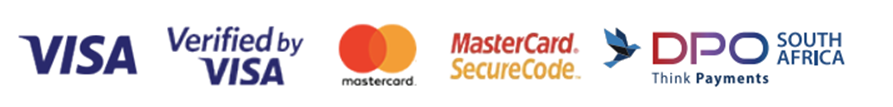﻿ Remediation & Enrichment Primary Maths Material (2) - Singapore Maths

per page
Showing 41 - 46 of 46 items
• ##### Conquer Mathematics (Factors, Multiples, Fractions) Book 4
R220.00

Designed to equip students with basic skills to solve questions involving factors, multiples and fractions. Book contains exercises that consist of multi-choice and short-answer questions. The exercises are diagnostic in nature.

• ##### Conquer Mathematics (Decimals and Time) Book 4
R220.00

Designed to equip students with basic skills to solve questions involving decimals and time. Book contains exercises that consist of multi-choice and short-answer questions. The exercises are diagnostic in nature.

• ##### Conquer Mathematics (Geometry, Area and Perimeter, Money, Graphs) Book 4
R275.00

Designed to enhance students' proficiency in mathematics concepts of geometry, area, perimeter, money and graphs. Book contains exercises that consist of multi-choice and short-answer questions. The exercises are diagnostic in nature.

• ##### Conquer Mathematics (Fraction, Decimal, Percentage, Ratio) Book 5
R220.00

Designed to enhance students' proficiency in mathematics concepts of fractions, decimals, percentages and ratios. Book contains exercises that consist of multi-choice and short-answer questions. The exercises are diagnostic in nature.

• ##### Conquer Mathematics (Measurements, Area and Volume) Book 5
R220.00

Designed to enhance students' proficiency in mathematics concepts of measurement, area and volume. Book contains exercises that consist of multi-choice and short-answer questions. The exercises are diagnostic in nature.

• ##### Conquer Mathematics (Fraction, Percentage, Ratio) Book 6
R250.00

Designed to enhance students' proficiency in mathematics concepts of fractions, percentages and ratio. Book contains exercises that consist of multi-choice and short-answer questions. The exercises are diagnostic in nature.

Showing 41 - 46 of 46 items